## 7260 Reputation

10 years, 205 days

## MaplePrimes Activity

### These are answers submitted by nm

Why would you load LinearAlgebra and then at same time make call to linalg:- ?

Important: The linalg package has been deprecated. Use the superseding packages, LinearAlgebra

if you remove that then it works. It might have to do with how linalg works. I do dot know for sure, but this works.

To multiply these matrices/vectors, just use the dot. So intead of what you had

linalg:-multiply(Ts_sksul, XCin)

you can just do

Ts_sksul.XCin

fixed.mw

diff(x^n,x) does not equal n*x^(n-1), but rather 0 if n=1

you probably meant rather 1, and not rather 0..

This seems like a bug to me

it is not a bug. all CAS systems work on a general expressions, not specific values.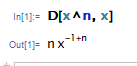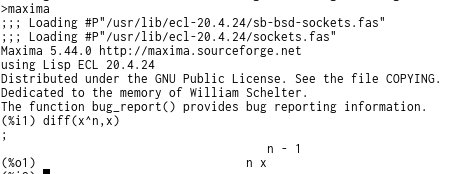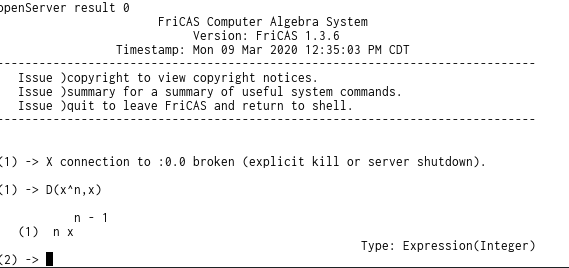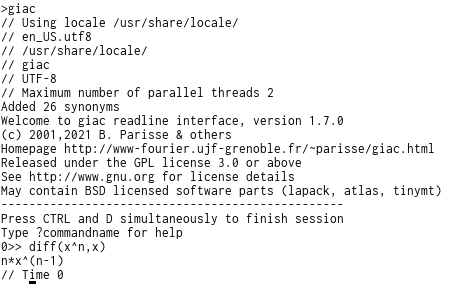To get derivative for specific you need to give specific value for n.

Maple by default uses 10 decimal points to display real numbers.

This works for 2021.1 on windows 10, using worksheet interface

```restart;
interface(typesetting=extended);
Typesetting:-Settings(striptrailing=true);
V := 4;
K := 16.56;
H := 0.5;
q1 := diff(y(x), x) = V*y(x)/H - K*y(x)/H;
```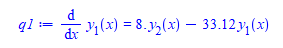Or you could do

```restart;
V := 4;
K := 16.56;
H := 0.5;
q1 := diff(y(x), x) = V*y(x)/H - K*y(x)/H:
convert(q1,rational)```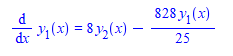See this post for reference. How-To-Delete-Zeros-After-Decimal-Point

When expression is   A+B then operands are A and B. And select selects operand which has what you wanted. So if the expression is t*u[1,1,1]*u[1,1,1,2]+2 then A=t*u[1,1,1]*u[1,1,1,2] and B=2, that is why it returned A.

When the input is t*u[1,1,1]*u[1,1,1,2] then expression is A*B*C and each one is now an operand. So A=t, B=u[1,1,1] and C=u[1,1,1,2] and that is why select now returned only u[1,1,1]

One way could be to do

```LT:=proc(expr, term)
if type(expr,`+`) or nops(expr)=1 or expr=term then
select(has, expr, term);
elif type(expr,`*`) then
if has(expr,term) then
return expr;
else
return NULL;
fi;
else
error "not expression?";
fi;
end proc;
```

And now it works for both cases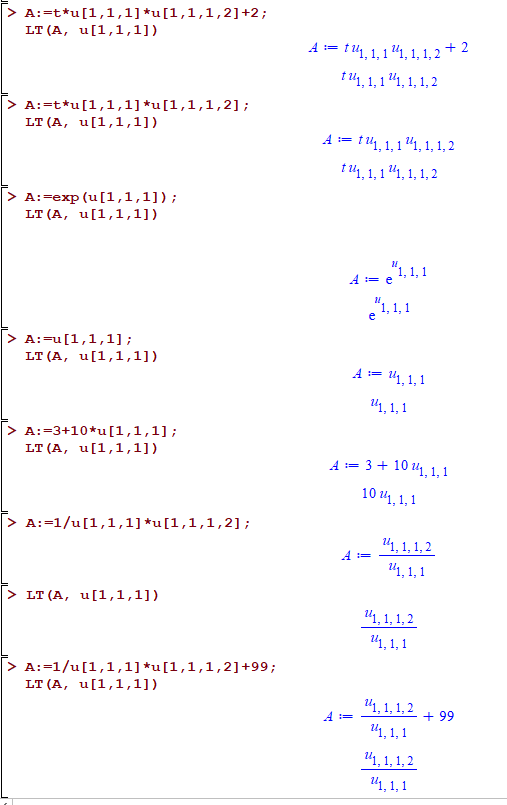may be

```restart;
ode1:=diff(x__1(t),t)=x__1(t)-x__2(t)-3;
ode2:=diff(x__2(t),t)=12*x__1(t)-8*x__2(t)+4;
vars:=[x__1(t),x__2(t)];
A,b:=LinearAlgebra:-GenerateMatrix([rhs(ode1),rhs(ode2)],vars):
sys:=Vector(diff(vars,t)) = A.Vector(vars)-b
```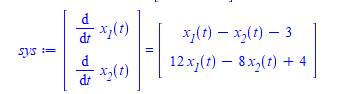`dsolve(sys)`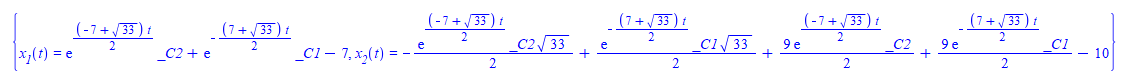ofcourse, you could just have done

`dsolve([ode1,ode2])`

And got same result.

edit

Is there another way to set up something from an equation to a matrix? More like an output like the above one?

I am not sure I understand exactly what the question here. May be you mean for display only purposes you want to change how it prints on the screen? You can try this line instead

```sys:=Vector(diff(vars,t)) = A %.Vector(vars)%- b
```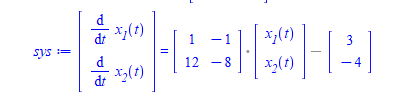Now it looks like the one you showed.

I do not use 2D math Maple laanguage at all. So not sure if this is what you want.

dsolve gives answer with integrals it could not evaluate. Try numerical

```restart;
sys := diff(x1(t), t) = 3*x1(t) - 2/1000*x1(t)*x2(t), diff(x2(t), t) = 6/10000*x1(t)*x2(t) - 1/2*x2(t);
ics := x1(0) = 1000, x2(0) = 500;
sol:=dsolve([sys,ics],numeric,{x1(t),x2(t)},output=listprocedure);
plot(rhs(sol)(t),t=0..4,labels=["t","x1"]);
plot(rhs(sol)(t),t=0..4,labels=["t","x2"]);

```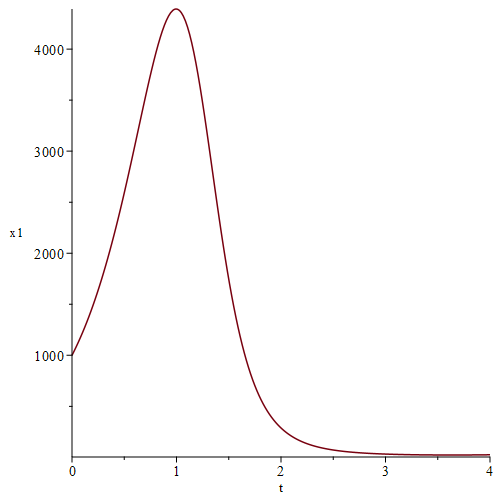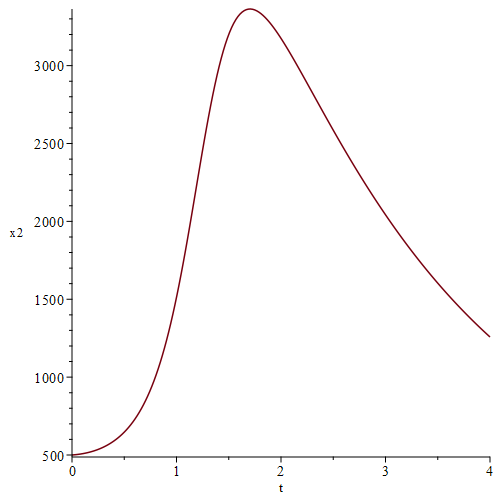one possibility could be

```restart;
G[i,k]:=u[i] - u[k]-(u[i]-u[k])^2;
```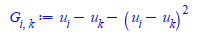```for i from 1 to 3 do
for j from 1 to 3 do
for k from 1 to 3 do
G[i,k]:=u[i] - u[k]-(u[i]-u[k])^2;
od;
od;
od;

G[2,1];
G[3,2];
```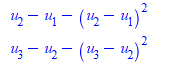its latex

```latex(G[2,3])

G_{2,3}```

double underscore could have worked  but do not know how to make it work with 2 indices (i,j).

For example , for the RHS you could do

`u__i- u__k-(u__j-u__k)^2`

But this would not work for G with double index.

```restart;